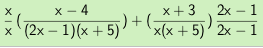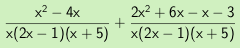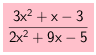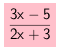### Home > CAAC > Chapter 12 > Lesson 12.4.2 > Problem12-77

12-77.
1. Add or subtract the following rational expressions. Then simplify your solutions, if possible. Homework Help ✎

1.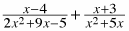2.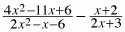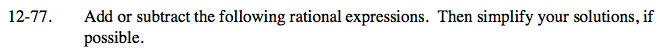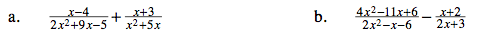Factor the denominators.

$\frac{x - 4}{(2x - 1)(x + 5)} \; + \; \frac{x + 3}{x( x+ 5)}$

Multiply the fractions by a form of one to get a common denominator.